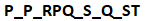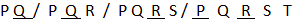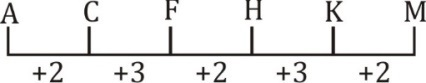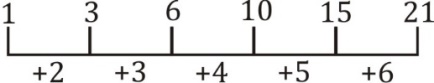Latest SSC jobs   »   Analogy and Series Reasoning Quiz for...

# Analogy and Series Reasoning Quiz for SSC CGL Exam 2020: 6th February 2020

Direction (1-3): In the following question, select the related words/letters /numbers from the given alternatives.

Q1. Book : Pages : : Ladder : ?
(a) Steps
(b) Walking
(c) Parts
(d) Levels

Q2. WTQ : DGJ :: NKH : ?
(a) MPS
(b) LOR
(c) NQT
(d) ORV

Q3. 11 : 1331 : : 12 : ?
(a) 1728
(b) 728
(c) 1528
(d) 1628

Q4. In the following question, which one set of letters when sequentially placed at the gaps in the given letter series shall complete it?(a) QRQPR
(b) QQRPR
(c) PQPRR
(d) PRQRQ

Q5. 3, 17, 73, 297, ?
(a) 1087
(b) 1193
(c) 2117
(d) 2197

Q6. MP, OR, QT, SV, ?
(a) UX
(b) TW
(c) TU
(d) XU

#### SSC CGL | SSC CHSL 2020 | Blood Relation Reasoning Tricks | Marathon Class | Part -2

Direction (7-10): A series is given, with one word missing. Choose the correct alternative from the given ones that will complete the series.

Q7. 0, 3, 8, ? , 24, 35
(a) 15
(b) 16
(c) 18
(d) 9

Q8. A, C, F, H, K, ?
(a) M
(b) N
(c) L
(d) O

Q9. 1, 3, 6, 10, 15, ?
(a) 20
(b) 21
(c) 22
(d) 24

Q10.If 6 @ 4 @ 7 = 101 and 2 @ 5 @ 11 = 150, then what is the value of A in A @ 8 @ 9 = 289?
(a) 5
(b) 8
(c) 12
(d) 17

#### Solutions:

S1. Ans. (a);
Sol. Book have pages. Ladder have steps.

S2. Ans. (a);
Sol. Pair of opposite letters.

S3. Ans. (a);
Sol.
11³ = 1331
12³ = 1728

S4. Ans. (b);
Sol.S5. Ans. (b);
Sol.
3 × 4 + 5 = 17
17 × 4 + 5 = 73
73 × 4 + 5 = 297
297 × 4 + 5 = 1193

S6. Ans. (a);
Sol. +2 series

S7. Ans. (a);
Sol.
0+3=3
3+5=8
8+7=15
15+9=24
24+11=35

S8. Ans. (a);
Sol.S9. Ans. (b)
Sol.S10. Ans. (c);
Sol.
6² + 4² + 7² = 101
2² + 5² + 112 = 150
12² + 8² + 9² = 289

You may also like to read:

#### Congratulations!General Awareness & Science Capsule PDF